# PRODUCT OF VECTORS INTERMEDIATE FIRST YEAR 1A CHAPTER 5 PROBLEMS WITH SOLUTIONS

Mathematics 1A Intermediate Product of vectors some problems with solutions are given below for examination purpose.

These are very simple to understand.

Study the textbook lesson Product of Vectors very well.

Observe the example problems and solutions given in the textbook.

You can also see

SSC Maths text book Solutions class 10

Inter Maths 1A Solutions textbook

Inter Maths 1B textbook solutions

Inter Maths 1IA text book solutions

Inter Maths IIB text book solutions

Product of Vectors

Exercise 5(a)

Exercise 5(b)

Exercise 5(c)

M

## VECTOR ALGEBRA PRODUCT OF VECTORS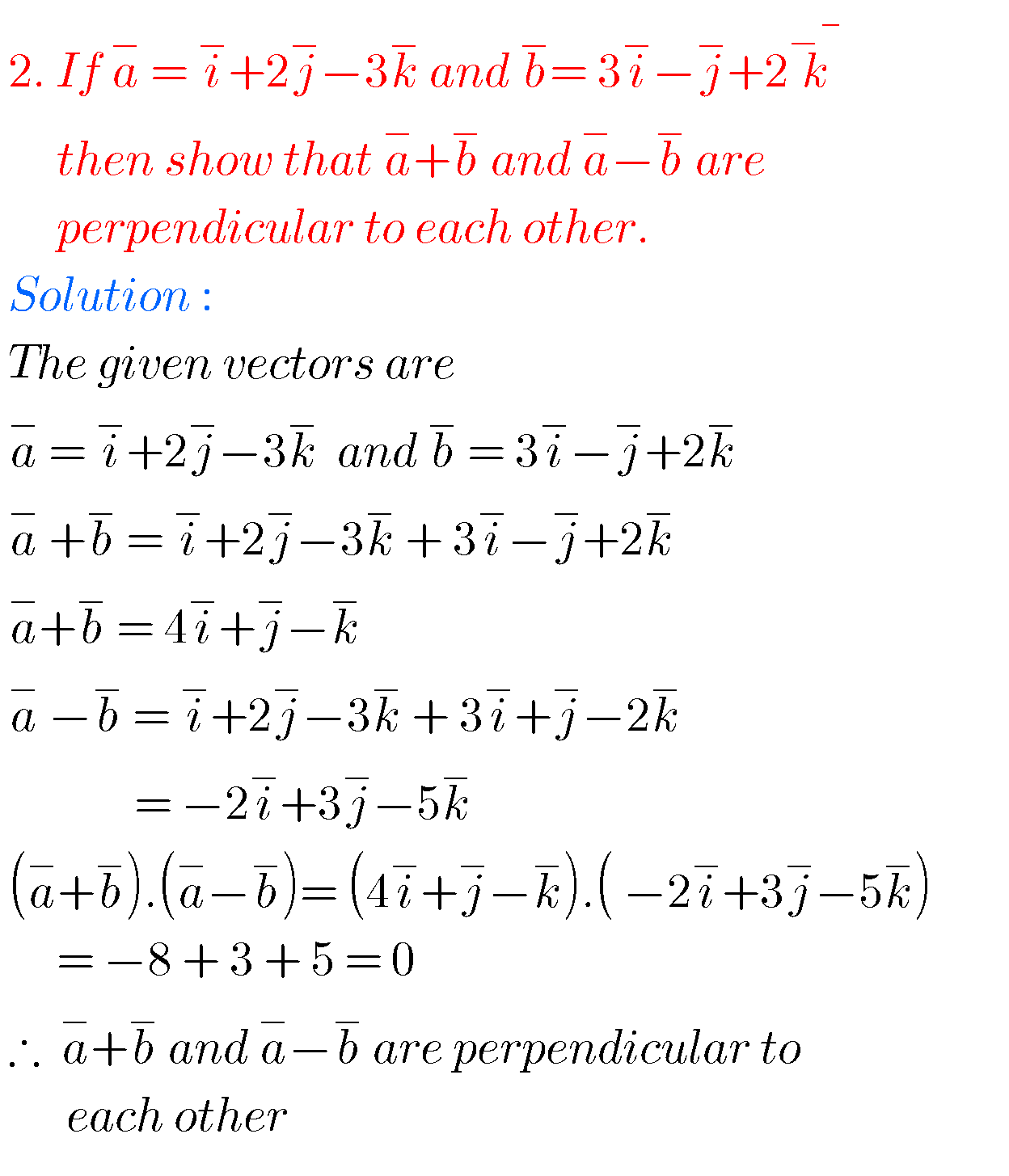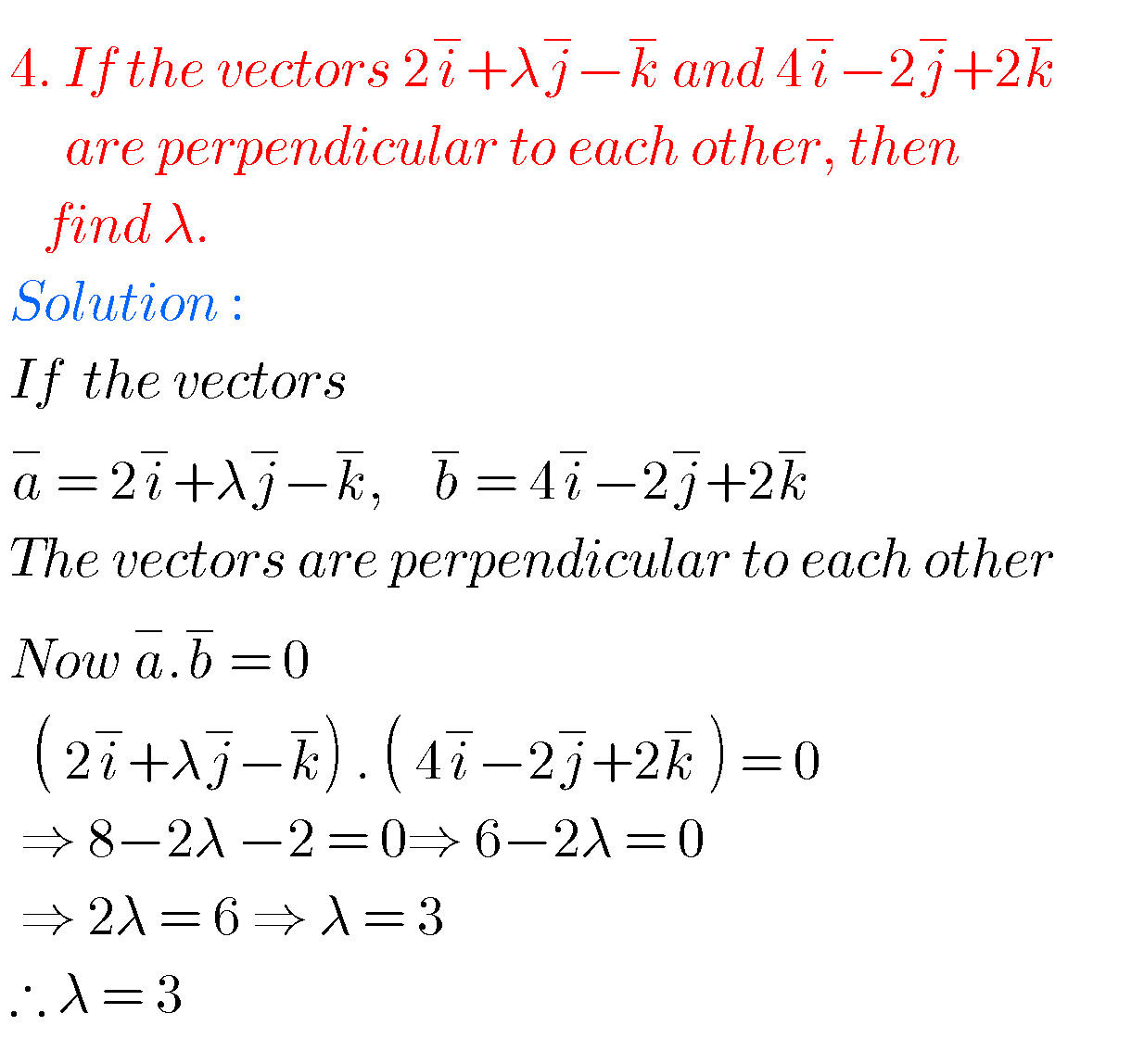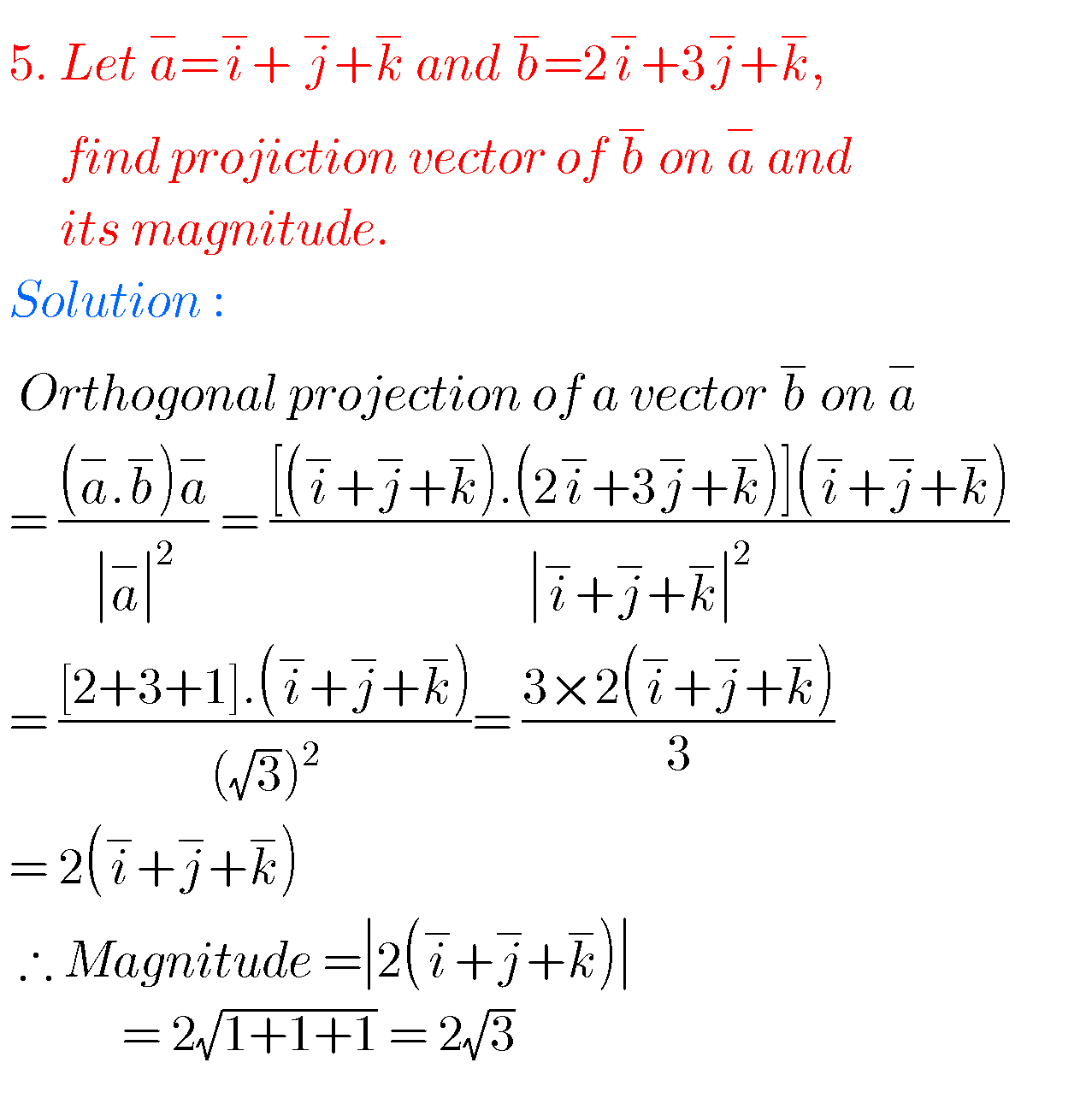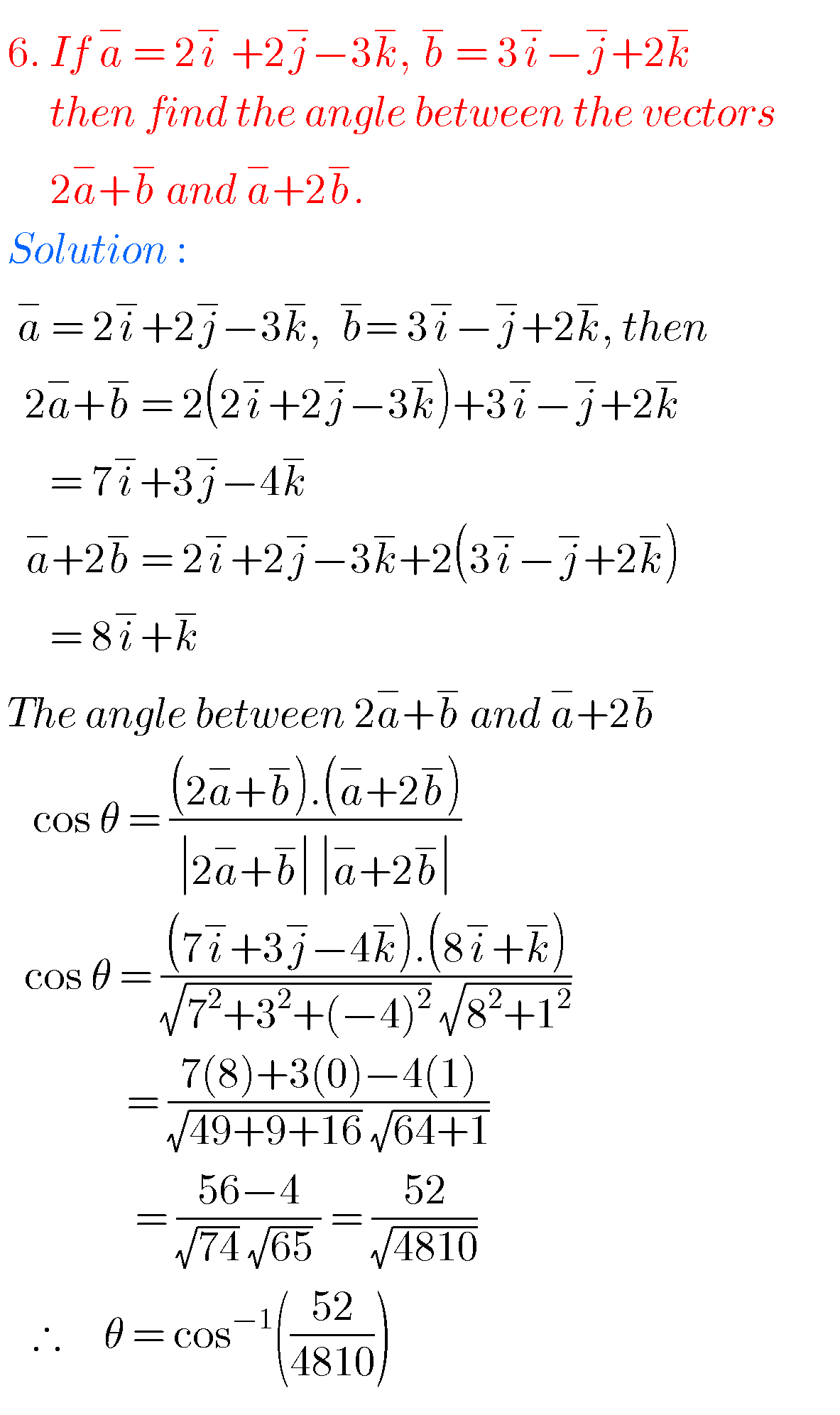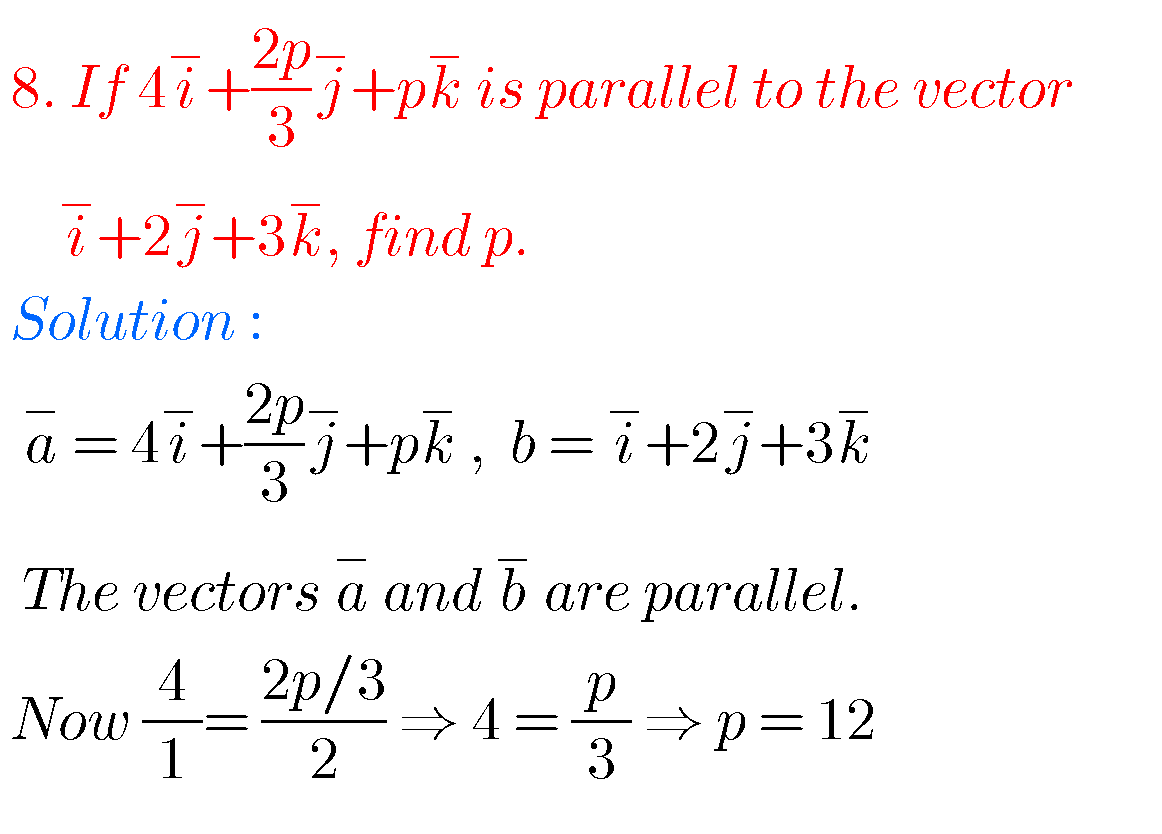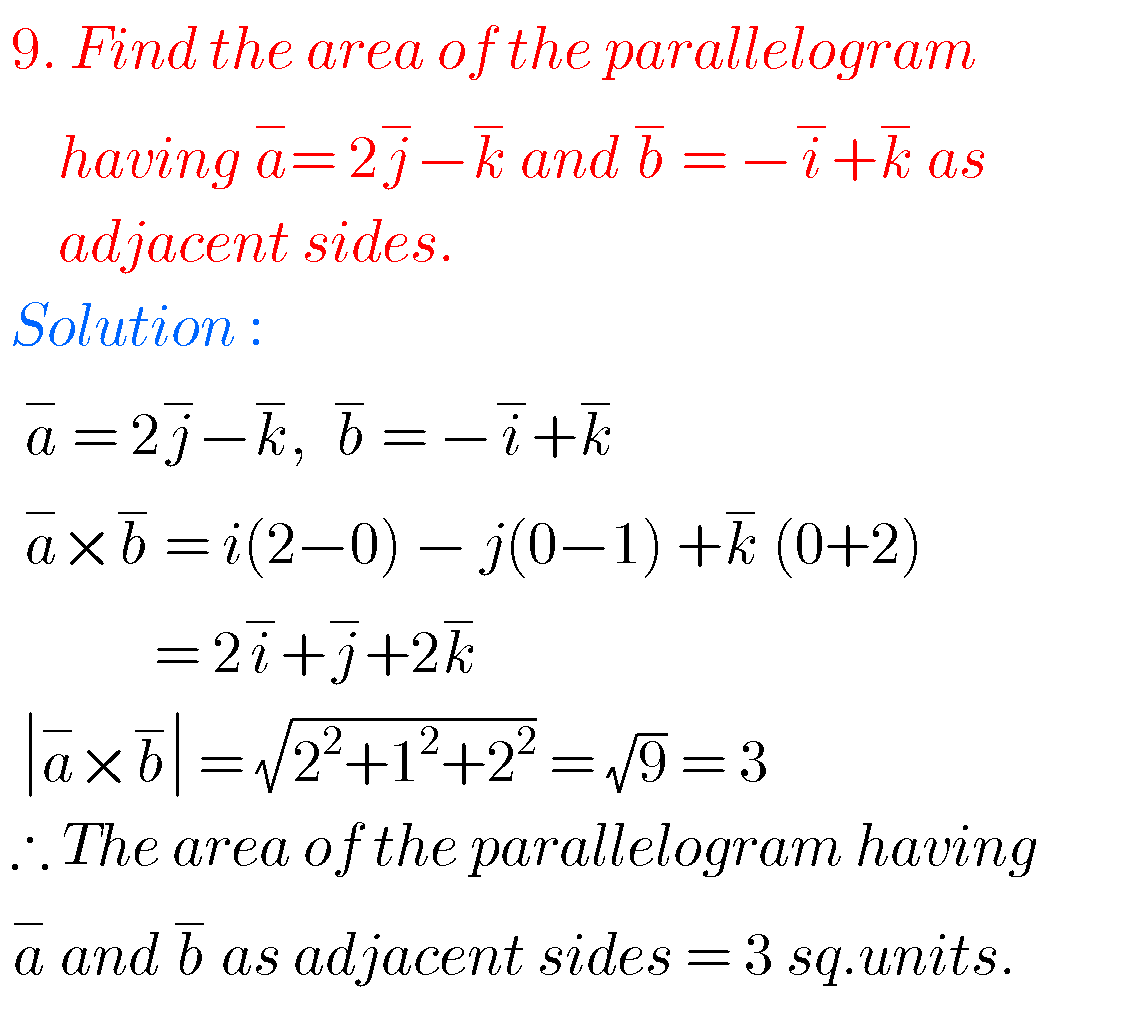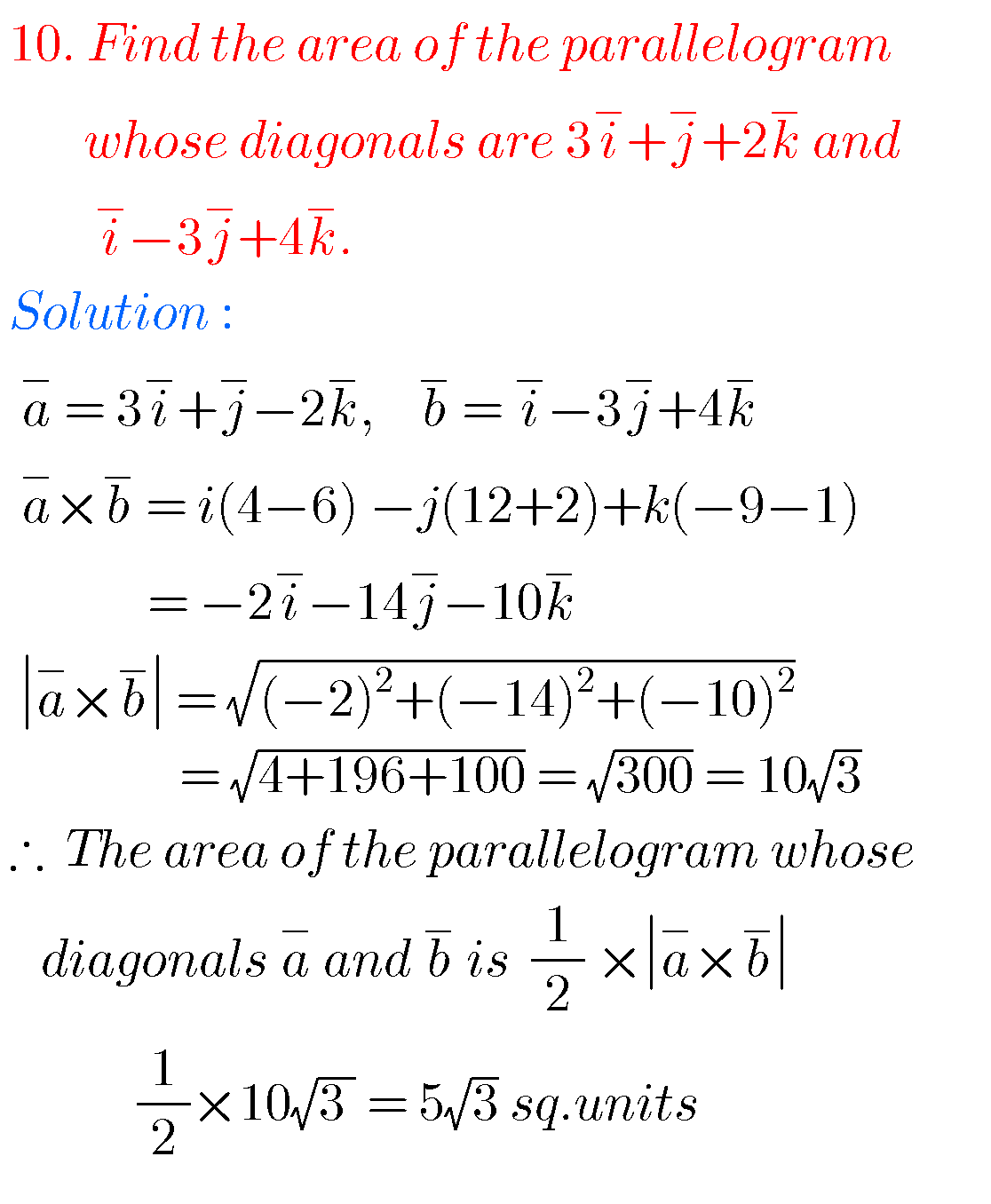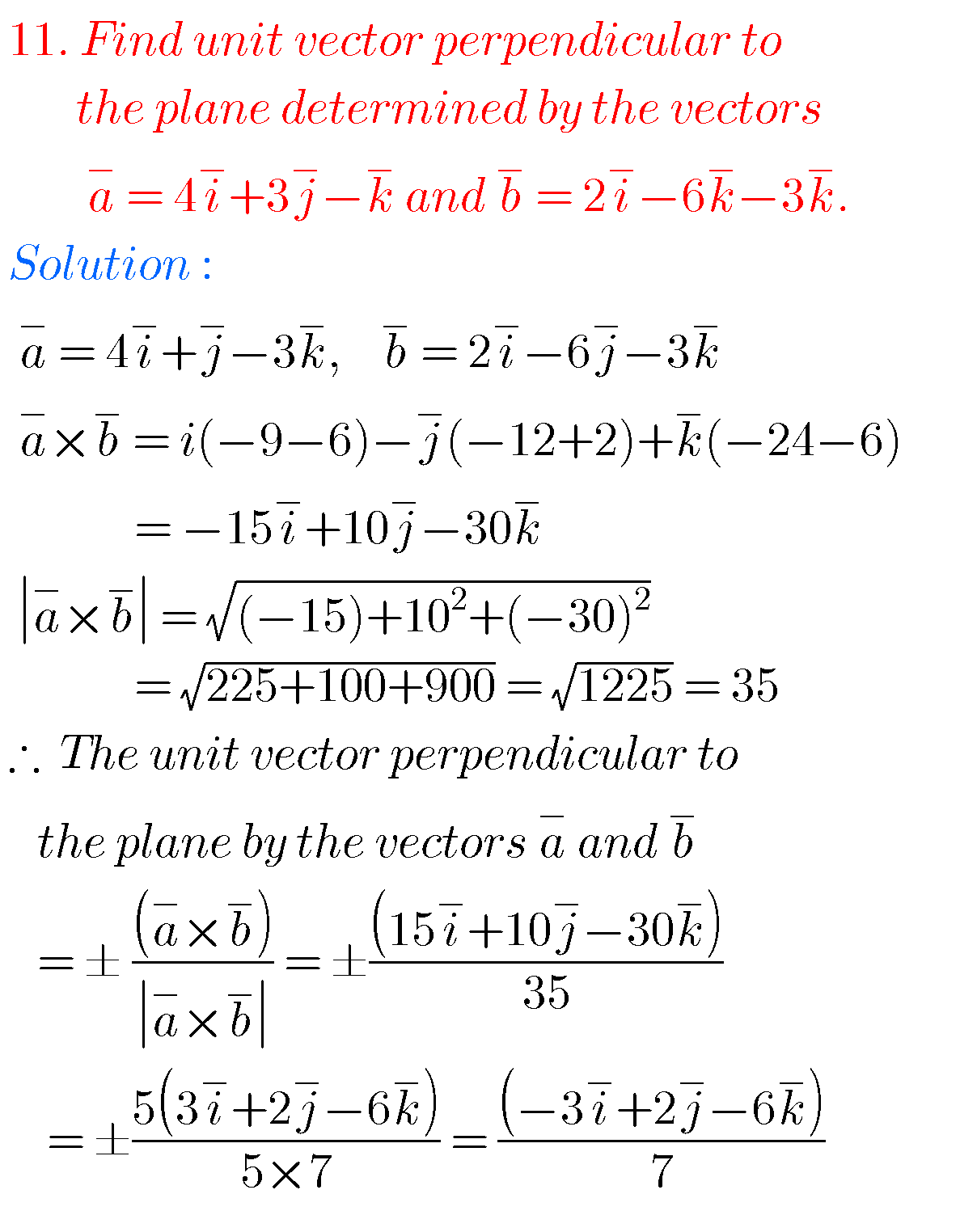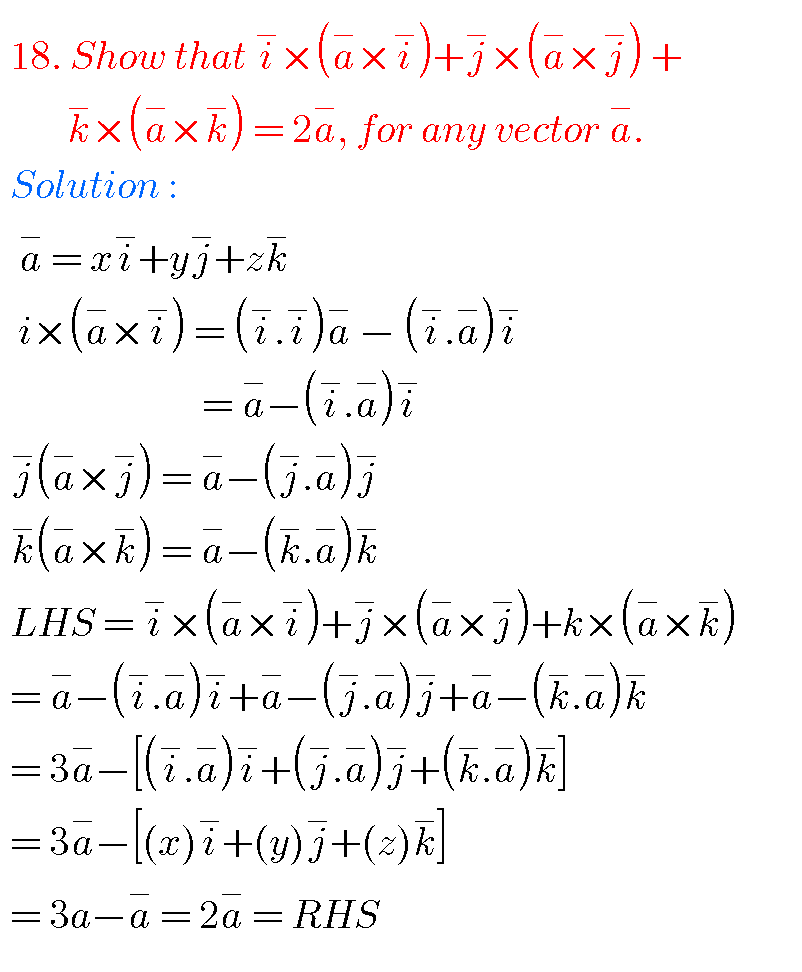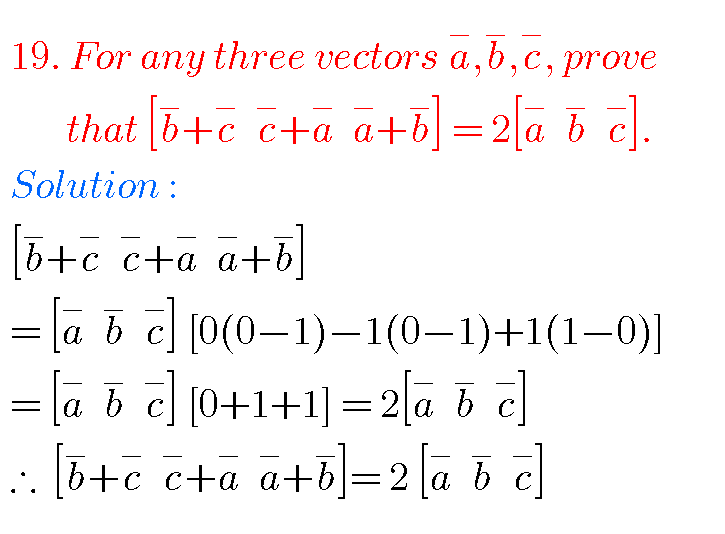### SOLUTIONS FOR JUNIOR INTER MATHS PRODUCT OF VECTORS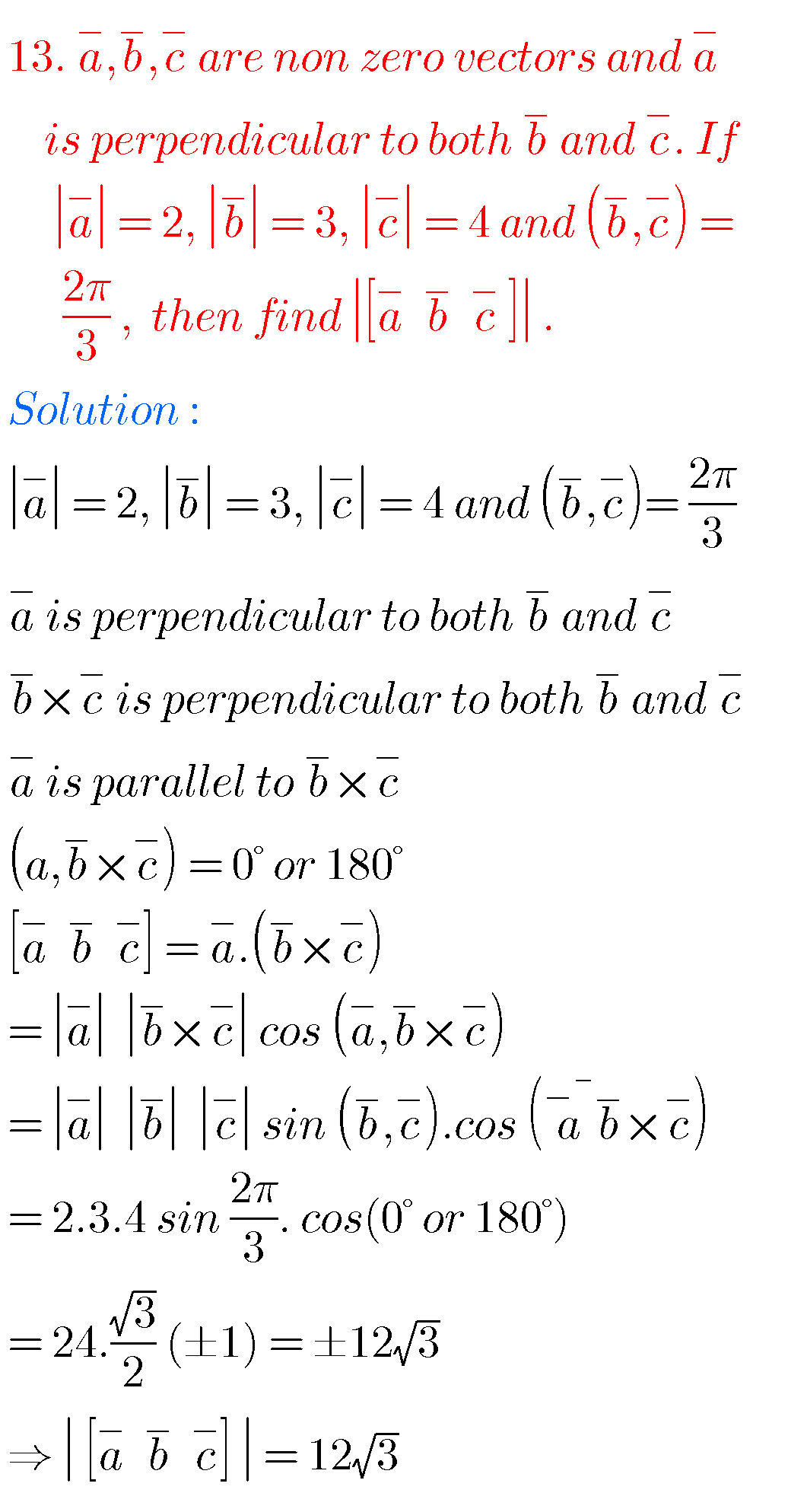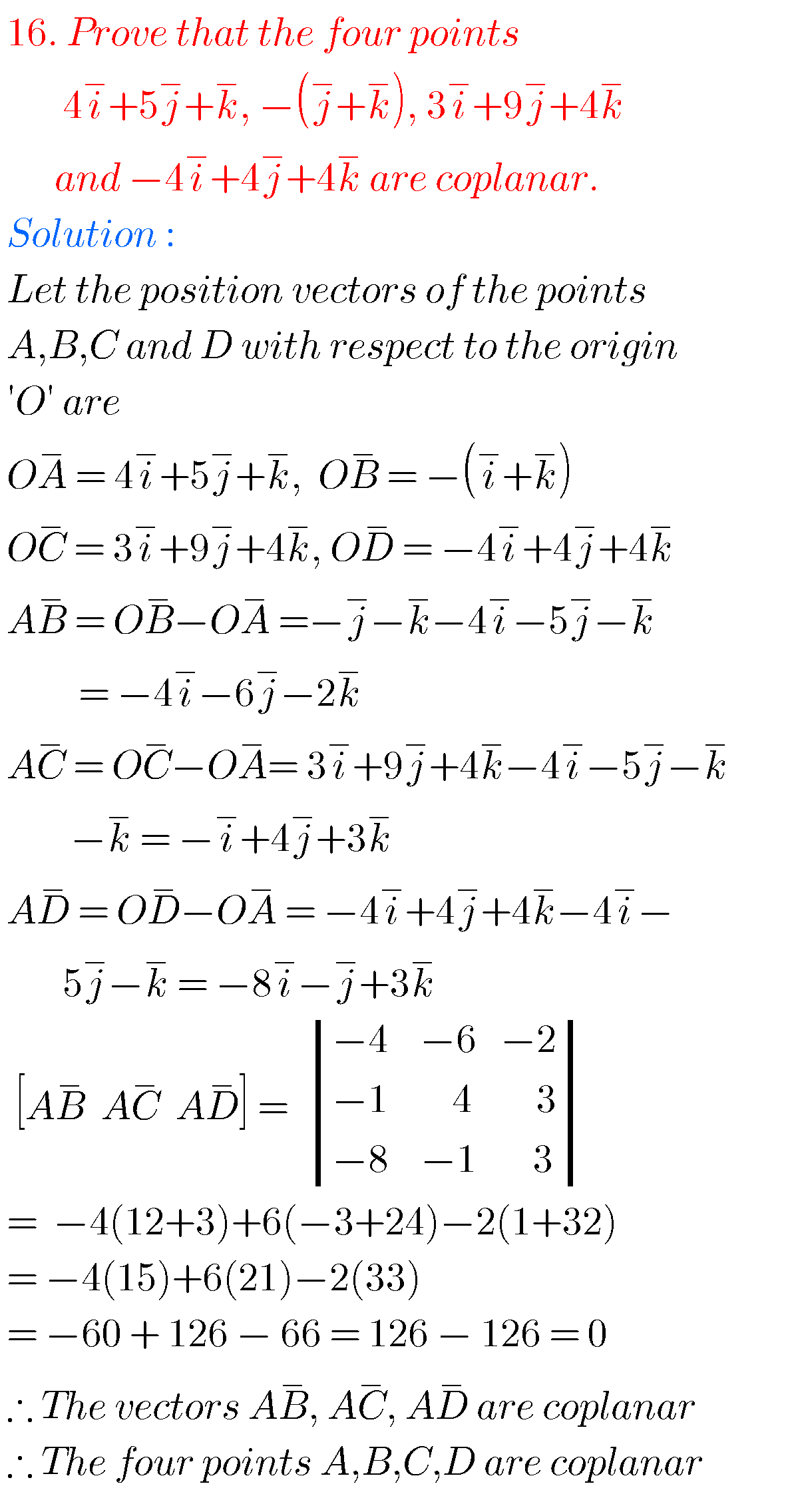Product of vectors long answer questions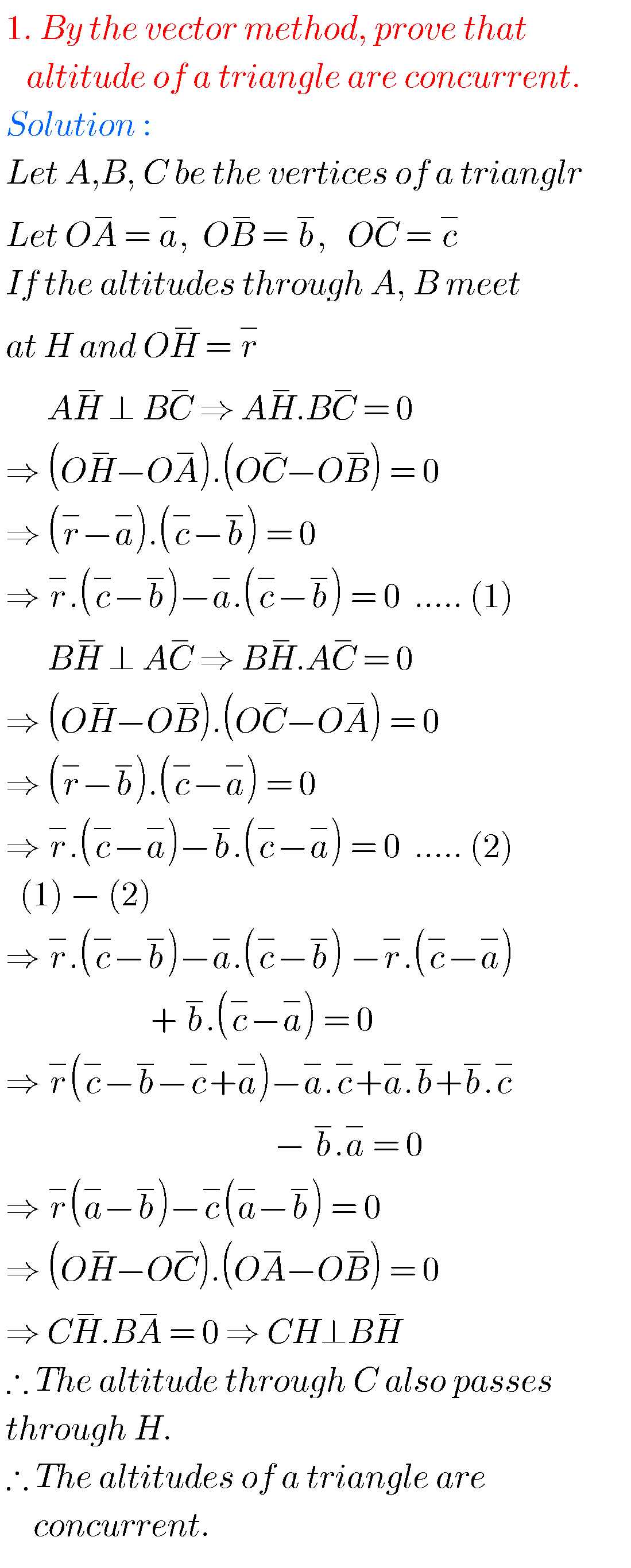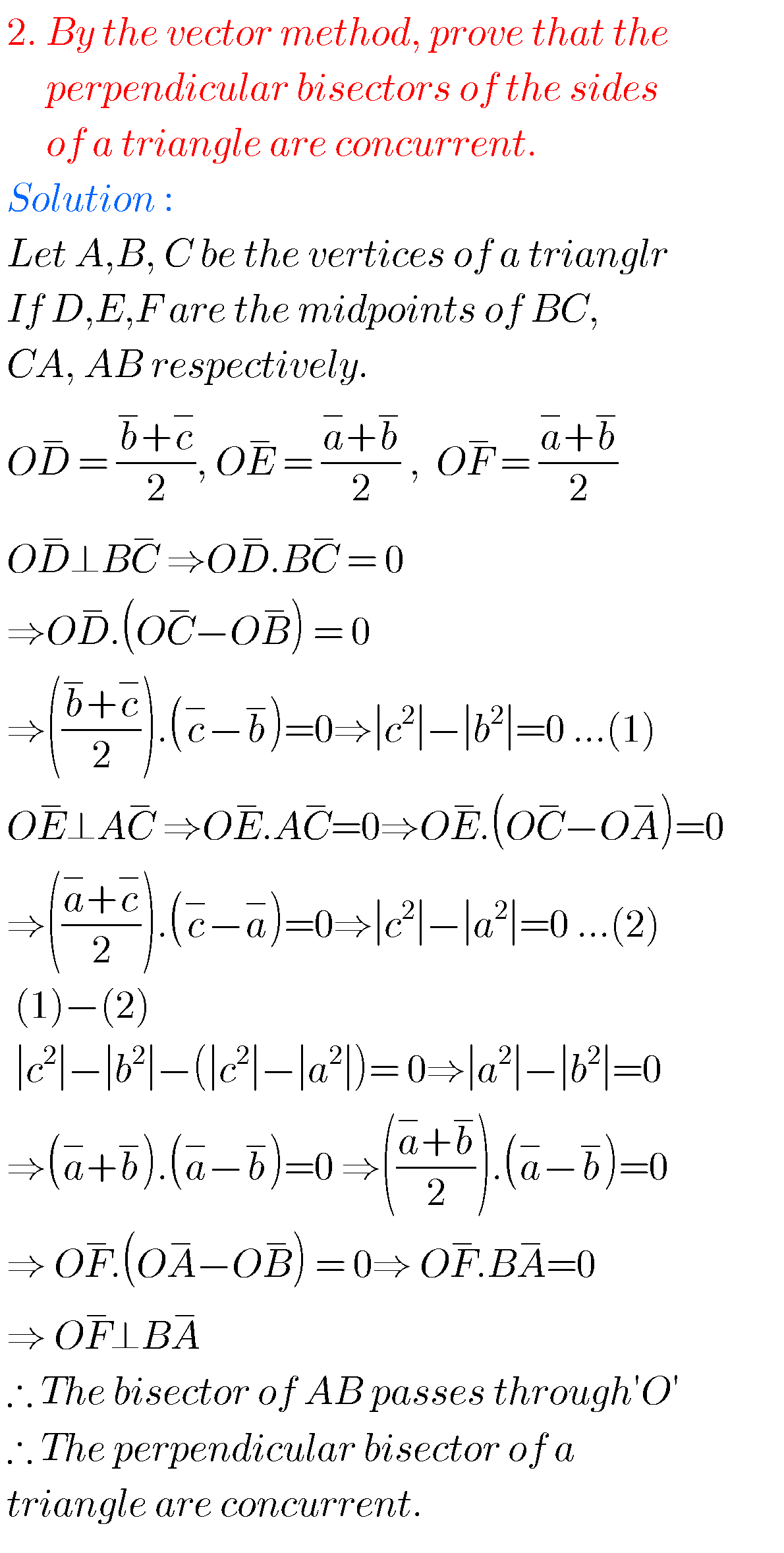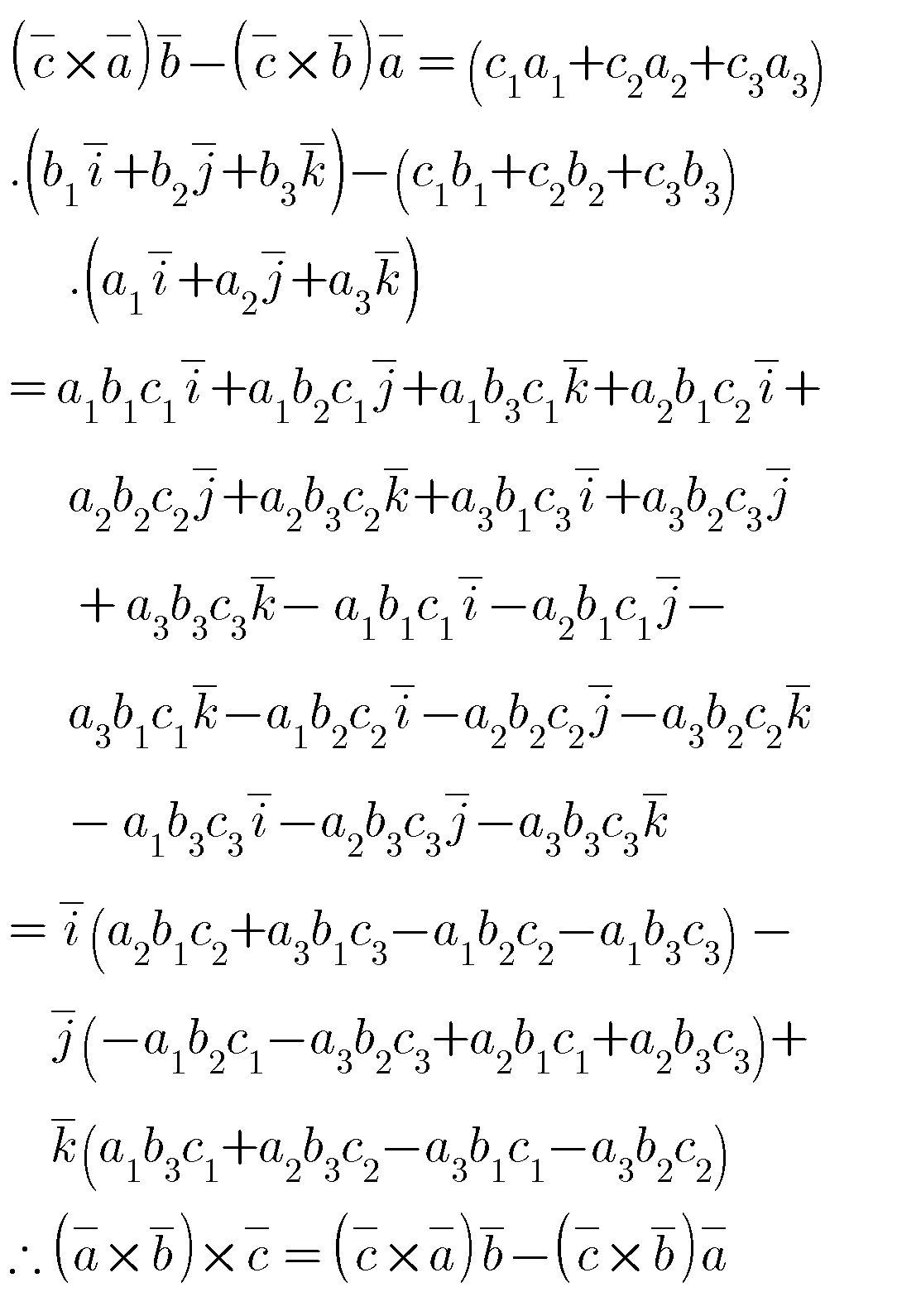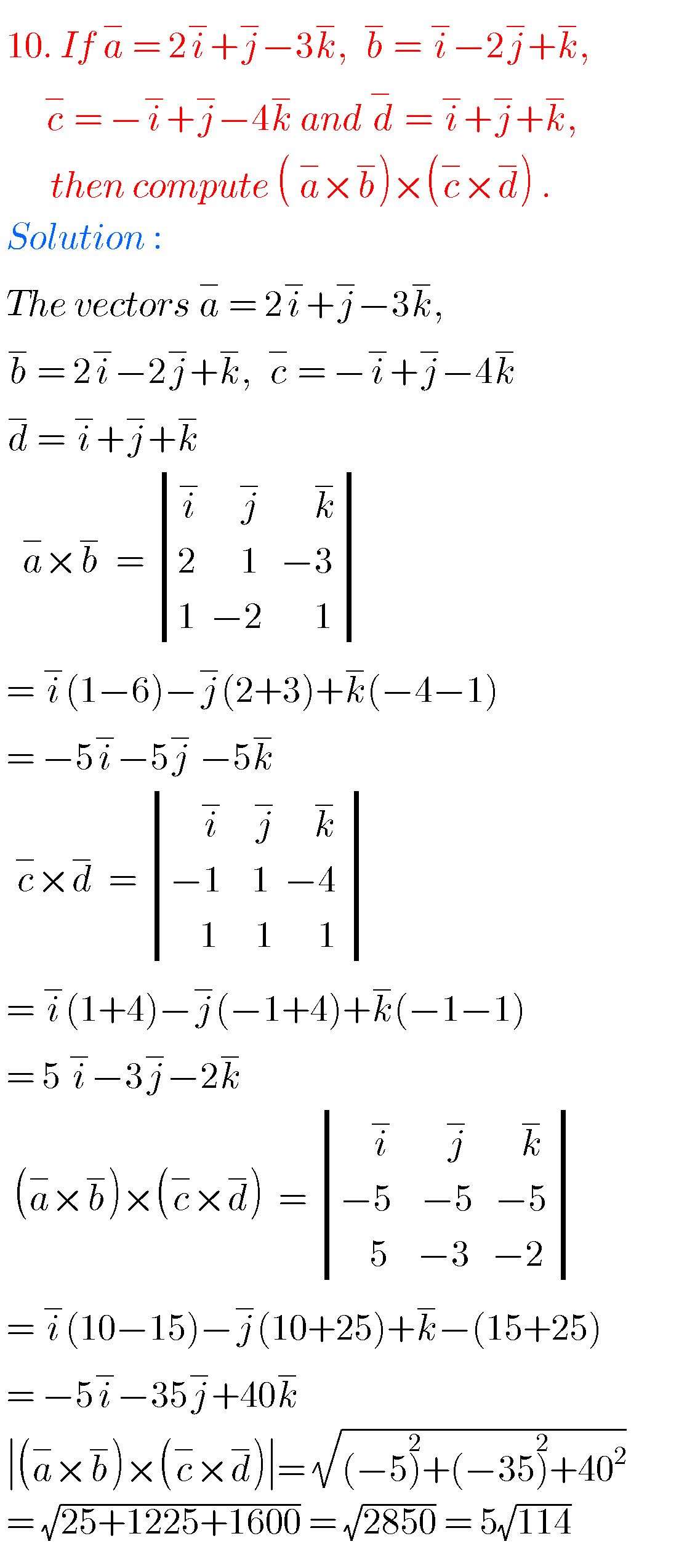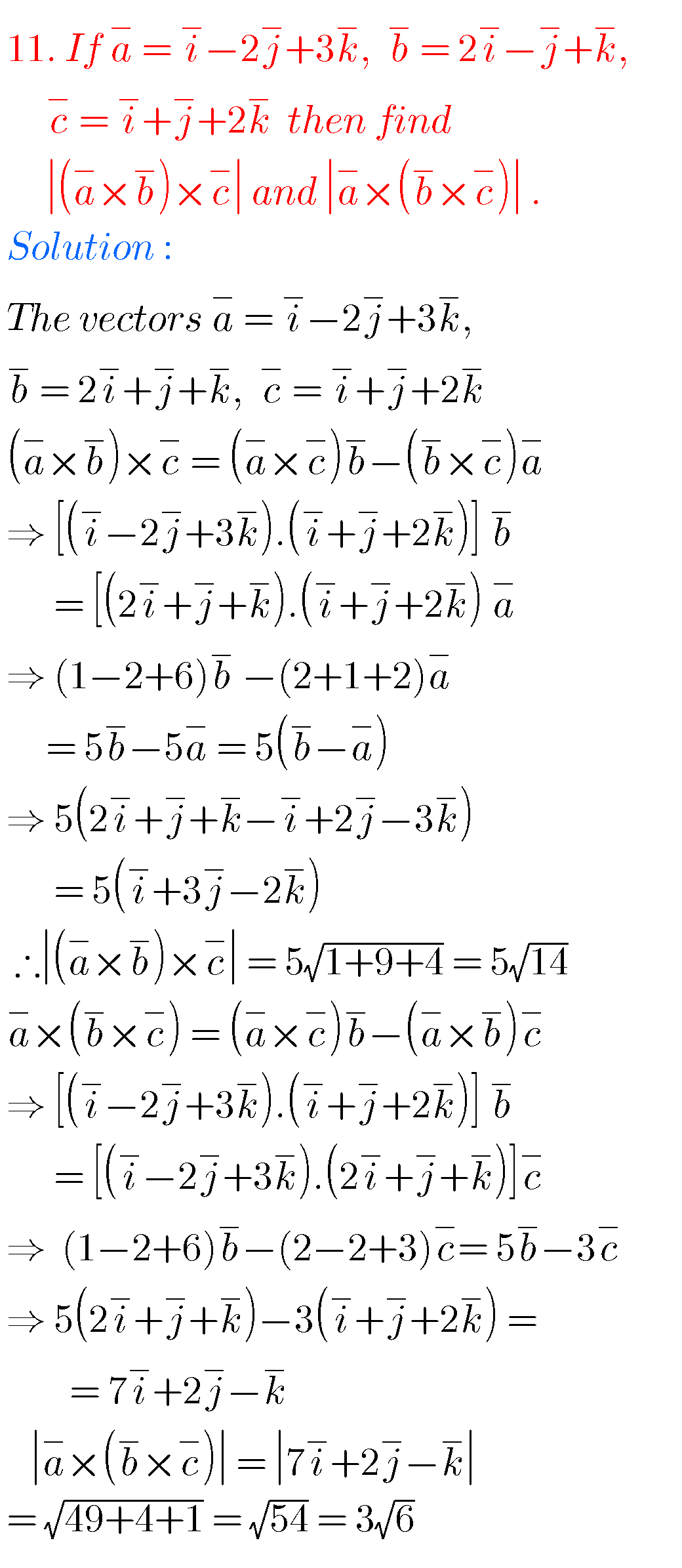Note : Observe the solutions and try them in your own methods.

Some more solutionsYou can see the solutions for junior inter maths 1A for examination purpose

3. Matrices

Exercise 6(a) inter maths 1A solutions

Exercise 6(b) inter maths IA solutions

Inter maths trigonometry solutions

### 2 thoughts on “Product of Vectors,Intermediate first year 1A chapter 5 problems with solutions”

1.I like this so much but it is better to improve it with all exercise sums

2.Wow it is very useful